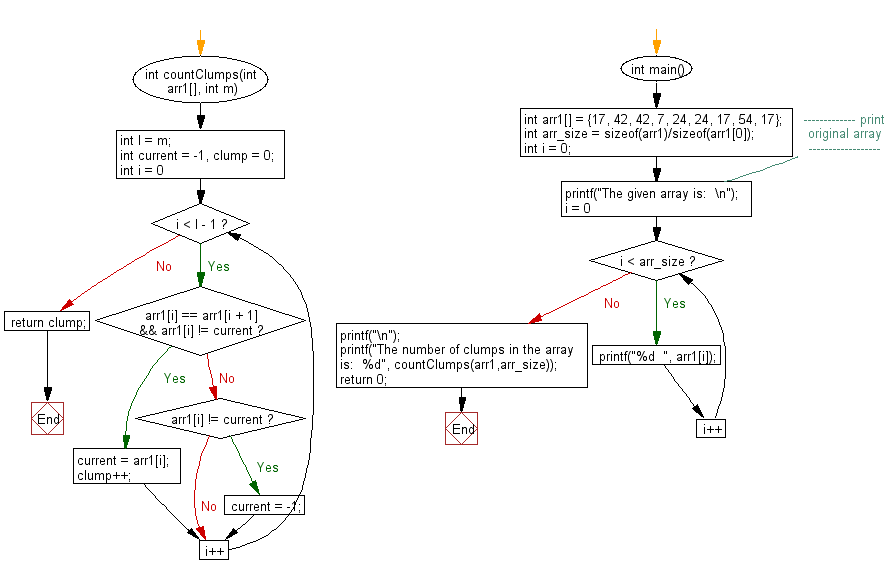﻿ C exercises: Return the number of clumps in a given array - w3resource# C Exercises: Return the number of clumps in a given array

## C Array: Exercise-100 with Solution

Write a program in C to return the number of clumps(a series of 2 or more adjacent elements of the same value) in a given array.

Sample Solution:

C Code:

``````#include<stdio.h>

int countClumps(int arr1[], int m)
{
int l = m;
int current = -1, clump = 0;
for(int i = 0; i < l - 1; i++)
{
if(arr1[i] == arr1[i + 1] && arr1[i] != current)
{
current = arr1[i];
clump++;
}
else
{
if(arr1[i] != current)
{
current = -1;
}
}
}
return clump;
}
int main()
{
int arr1[] = {17, 42, 42, 7, 24, 24, 17, 54, 17};
int arr_size = sizeof(arr1)/sizeof(arr1);
int i = 0;
//------------- print original array ------------------
printf("The given array is:  \n");
for(i = 0; i < arr_size; i++)
{
printf("%d  ", arr1[i]);
}
printf("\n");
//-----------------------------------------------------------
printf("The number of clumps in the array is:  %d", countClumps(arr1,arr_size));
return 0;
}
```
```

Sample Output:

```The given array is:
17  42  42  7  24  24  17  54  17
The number of clumps in the array is:  2
```

Pictorial Presentation:Flowchart:C Programming Code Editor:

Improve this sample solution and post your code through Disqus.

﻿

## C Programming: Tips of the Day

Where is the C auto keyword used?

auto is a modifier like static. It defines the storage class of a variable. However, since the default for local variables is auto, you don't normally need to manually specify it.

Ref : https://bit.ly/3yzwC9r